## Ratio

The ratio of two numbers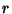and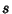is written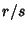, whereis the Numerator andis the Denominator. The ratio oftois equivalent to the Quotient. Betting Odds written as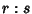correspond to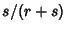. A number which can be expressed as a ratio of Integers is called a Rational Number.